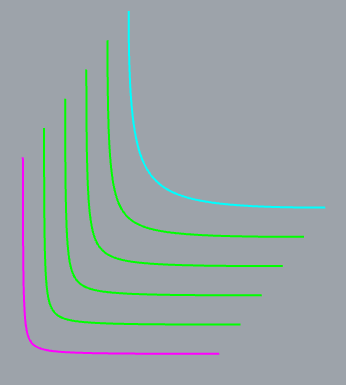# Tweening "conic" curves has unexpected results

I made two curves by making two rounded rectangles with conic corners and then just taking the lower left corners by splitting at the midpoints of the left and bottom sides.

I got this

Then I tried TweenCurves between magenta and cyan and got this

For me, this was quite unexpected. I thought that I would see something like this

Is this a surprise to you Rhino devs?

Is this a bug?

What is the cause?

Is there a way to get what I want via some other method?

Could TweenCurves be changed to cope with curves like mine?

I have attached a 3dm file to demonstrate.

tweenConicCurves.3dm (28.1 KB)

The curves have three control points. I drew three red lines between the corresponding points and divided them into the number of curves that will be generated.

I got this

The control points at the ends of the lines are what you would expect but the middle ones are definitley wrong. The closer a curve is to the cyan end curve, the further its middle control point is away from where you would expect it to be.

See?

I manually moved them and got something close to acceptable.

I think that the curve below the cyan end curve is too far out. And, maybe, the curve immediately after the starting magenta curve is a bit wrong too.

I suspect that you need access to the code to fix this.

Have funI have uploaded another 3dm file. Click on each curve to see where its middle control point is.

tweenConicCurves-controlpoints.3dm (37.8 KB)

The distance between the 3 points of the curve created with Tween are not the same distance apart, while the original curve control points are the same.

I have to conclude that’s a bug.

https://mcneel.myjetbrains.com/youtrack/issue/RH-72273/TweenCurves-creates-skewed-result

Thanks JohnIf the two end points are on top of each other then all the, corresponding, control points are on top of each other yet the curvatures in the middle are different so I assume that the only difference is a weighting applied to the middle point.

Maybe that weighting has to be lerpped as well in order to see correct results. (or lerpped correctly). I could change the position by dragging but not the weight.

I would expect the weighting to be changed with Tweening.
What I don’t understand is why the middle point in the Tweened curve is not in line with the original input curves.

The legs of the tweened curve are not equidistant, while the legs of the input curves are.
That seems wrong.

Conic curves in Rhino are rational NURBS. Rational means the control point weights are unequal. Most curves in Rhino are non-rational which means the all control point weights are equal (usually equal to 1).

TweenCurves struggles with rational input curves when MatchMethod=None is used. An alternatives is to use MatchMethod=Refit or MatchMethod=FitPoints. The results will be non-rational curves with potentially a large number of control points. Use Rebuild or FitCrv to reduce the number of control points. if possible within the accuracy needed.

Yeah, if you evenly space the middle point spacing and the weighting both, you can get something that looks correct.Probably scriptable…

-Pascal

result of
_loft
_divide
_extractIsoCurve

(i always thought, that s what tween is doing under the hood - but looks like i was wrong)

Creating a surface with Loft, and then extracting isocurves at equal intervals along the edges of the surface results in:

The resulting extracted isocurves are at equal intervals in parameter space, not physical space. Because the input curves used have equal weights at the corresponding ends equal intervals in physical space correspond to equal intervals in physical space at the ends. But the corresponding interior curves have different weights which results in the intervals in physical space in the interior being unequal intervals even though the intervals are equal in parameter space.

If both input curves to Loft are non-rational then all weights are 1 and equal. That results in equal intervals in parameter space corresponding to equal intervals in physical space.

The large number of control points makes me recoil in horror and I’m not even an class A surface modeller type.I prefer Pascal’s suggestion as I am extruding surfaces, then solids, and then doing solid intersections followed by filletEdges… so I want to be as light as possible.

My ideal world would be were TweenCurves just did the right thing.

Next best thing would be if I could, manually, change the weights (OR script it).

I’m not blocked by this because there is no requirement to use exact curves in precise positions. Even just manually moving the middle points gives me good enough results.

I’m interested in doing this in grasshopper. I boolean intersect solid “fins” made with these curves against a polysurface made from a subD with a star point on it (five edges converging on a point). Those edges can be baked into my intersected solids and that makes filletEdge tricky (needs manual fixup).

I’m hoping that i can use sliders in grasshopper to move the fins around and avoid the star edges.

So how would i edit the weights in the RhinoCommon API?

`Weight` command to change weights. I don’t know what the Grasshopper equivalent is.

@jmcauley - this script will (should) tween 3 point curves correctly… give it a spin, it might work.

TweenConics.py (4.6 KB)

To use the Python script use `RunPythonScript`, or a macro:

`_-RunPythonScript "Full path to py file inside double-quotes"`

-Pascal

Thanks Pascal, that was really usefulIt worked a treat.